Precalculus : Find the decimal value of a trigonometric function

Example Questions

Example Question #1 : Evaluating Trig Functions

Find the value of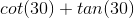to the nearest tenth if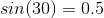and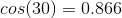.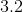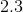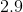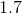Explanation:

Rewritein terms of sine and cosine.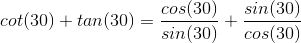Substitute the known values and evaluate.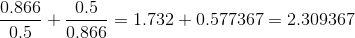The answer to the nearest tenth is.

Example Question #1 : Find The Decimal Value Of A Trigonometric Function

Determine the value of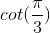in decimal form.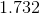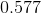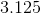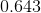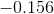Explanation:

Ensure the calculator is in radian mode since the expression shows the angle in terms of.  Also convert cotangent to tangent.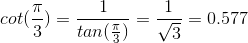Example Question #1 : Find The Decimal Value Of A Trigonometric Function

Find the decimal value of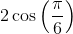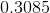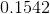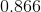Explanation:

To determine the decimal value of the following trig function,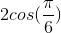, make sure that the calculator is in radian mode.

Compute the expression.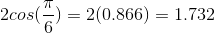Example Question #1 : Evaluating Trig Functions

Determine the correct value of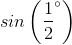.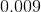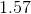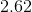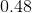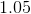Explanation:

The question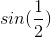asks for the y-coordinate on the unit circle when the degree angle is.

Be careful not to confuse finding the value of the angle when the y-value of the coordinate of the unit circle is.

Ensure that the calculator is in degree mode.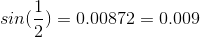Example Question #2 : Find The Decimal Value Of A Trigonometric Function

Find the value of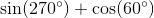.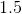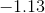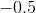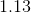Explanation:

Before beginning this problem on a calculator, though this is not necessary since these are special angles, ensure that the mode of the calculator is in degrees.

Input the values of the expression and solve.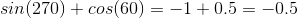All Precalculus Resources